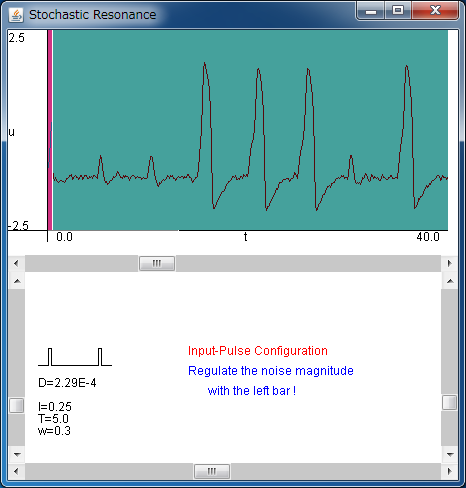# Stochastic ResonanceIf the above application does not start, please install OpenJDK from adoptium.net.

In this page, we treat "Stochastic Resonance", which is a phenomenon where a weak input signal is enhanced by its background noise. It is thought that the biological sensory system utilizes this phenomenon to detect emergent signals from outer environment.

FitzHugh-Nagumo equation with noise is written as

du/dt= c (- v + u -u3/3 + I(t) + s(t))
dv/dt= u - b v + a

where u is the membrane potential of the neuron, I(t) is an external input to the neuron, s(t) is its background noise, and a, b, and c are parameters. Note that we use the periodic pulse train as I(t), and we fixed parameters as a=0.7, b=0.8, and c=10.

The upper field of the applet shows the behavior of the membrane potential u. The horizontal axis denotes time, and the vertical one denotes u. With the default configuration, the model does not produce pulse because the strength of input pulse is too small.

The lower field of the applet shows the wave form of the periodic input pulse train I(t). With the right volume bar, you can regulate the strength of the input pulse.
And you can regulate the intensity of noise with the left volume bar. With the default configuration there is no noise in the system.

Let us inject the noise to the system by regulating the left volume bar. Unlike the case without noise, you can see the model generates pulses. If the intensity of noise is not so large, the output pulse almost synchronizes to the input pulse. However, with even larger intensity of noise, the output pulses will be generated randomly.

From these observations, we can see that there is an optimal noise intensity for the output pulse to response to the input pulse. This phenomenon is called as Stochastic Resonance.

In this applet, noise is added to the system, but it is known that Stochastic Resonance takes place in the noise-free chaotic system.

Moreover, when the parameter of the input is fixed as T=w=1, a constant input is injected to the model. When noise is added to such a system, the periodicity, or the coherence, of the system is known to be maximized at an optimal noise intensity. This pheonomenon is called coherence resonance. The role of coherence resonance in the biological system is still unknown.